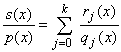#Interactive Real Analysis

Next | Previous | Glossary | Map

## 7.2. Integration Techniques

### Theorem 7.2.11: Partial Fraction Decomposition

Suppose p(x) is a polynomial of degree n such that p(x) =, where each qj is polynomial of degree j that is irreducible over R. If s(x) is another polynomial of degree less than n with no factors in common with p(x), then the rational function s(x) / p(x) can be written as a finite sumwhere each rj is a polynomial of degree less than j.

### Proof:

The proof is easiest when allowing complex numbers instead of reals and is not given.
Next | Previous | Glossary | Map